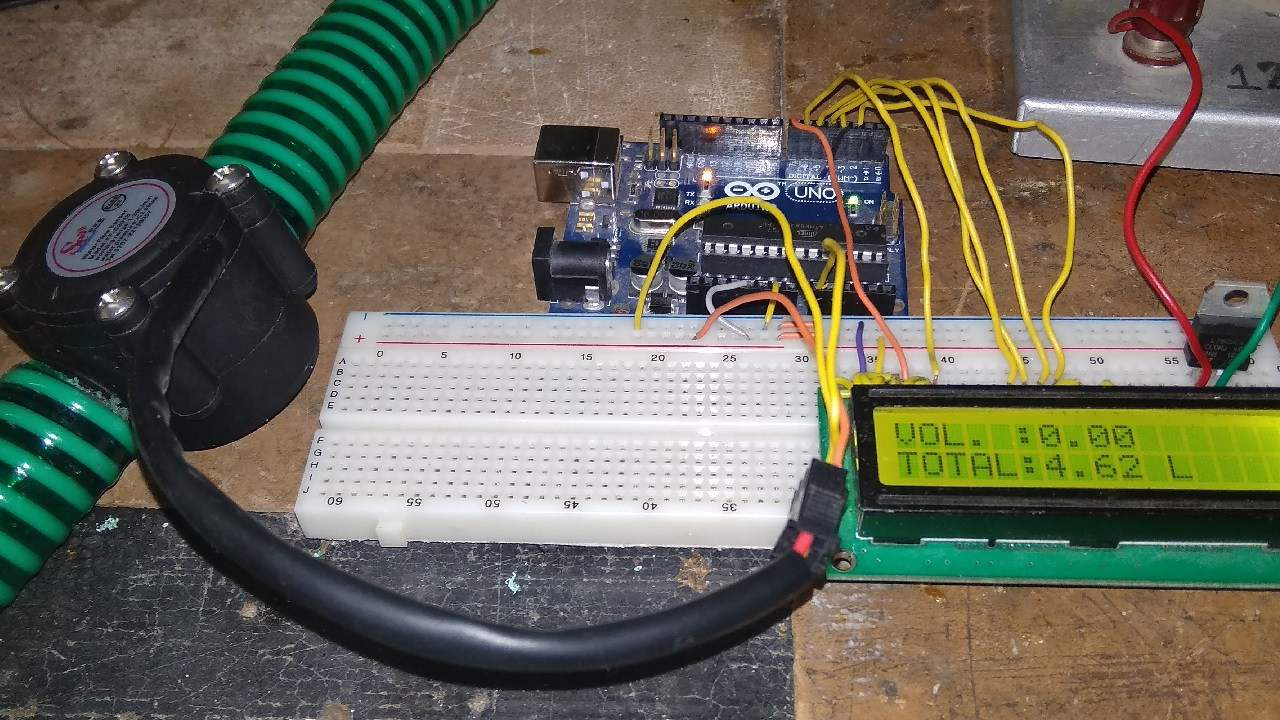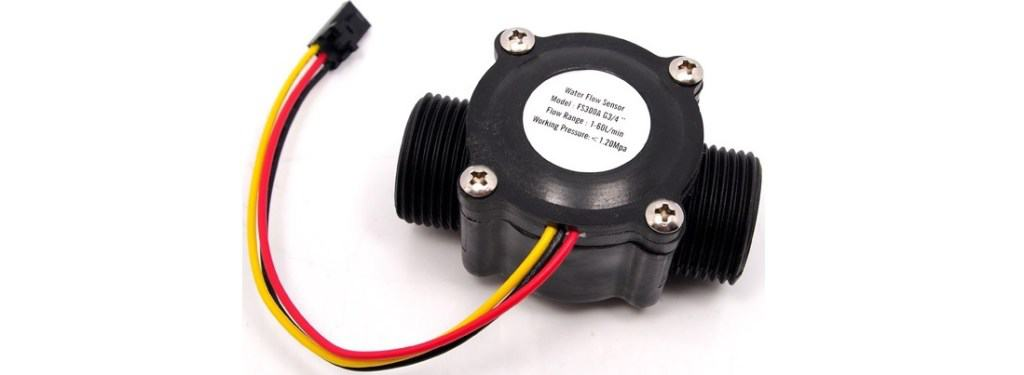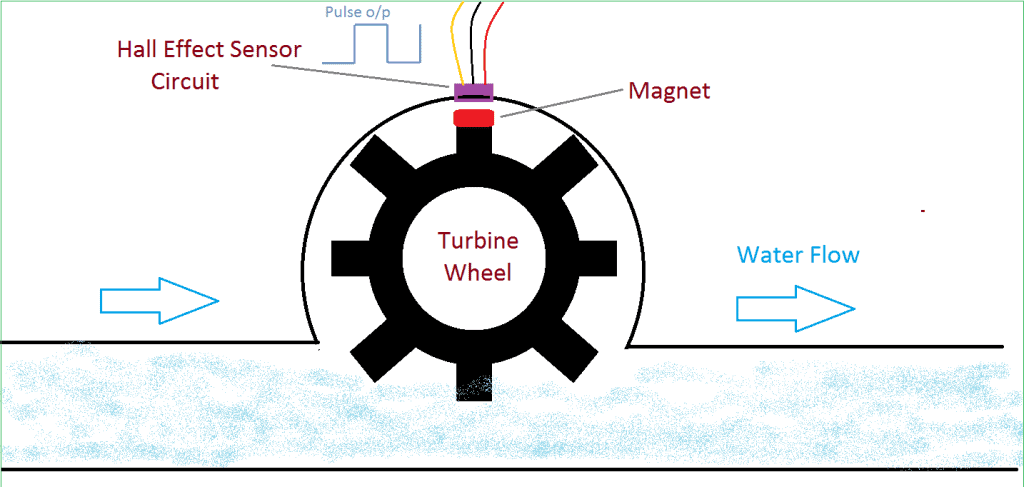Water Flow Sensor for Flow Rate & Volume Measurement using Arduino(Last Updated On: March 21, 2019)

Introduction:

In this project we will interface YFS201 Hall Effect Water Flow Sensor with Arduino for measuring flow rate and volume of water or any other liquid. This is a very wonderful project that can be used in industry or at home or at water flow measurement application in water tap, tunnel, river etc. Water Flow Sensor for Flow Rate & Volume Measurement using Arduino code along with ciruit diagram is explained below.

This project can be used to measure liquid flowing through a pipe or container, or to create a control system based on the water flow rate or quantity. For example, you could use this while gardening to measure the amount of water used to water your plants, to prevent wastage.

Water Flow Sensor for Flow Rate & Volume Measurement using Arduino:

Components Required:

```1. YFS201 Hall Effect Water Flow Sensor  (Buy Online)
2. Arduino Uno Board
3. 16 x 2 LCD Display
5. Connecting Wires
6. Mechanical pipe & Funnel (for poring water) shown in video below```

Circuit Diagram:YFS201 Hall Effect Water Flow Sensor:

Introduction:

This sensor sits in line with your water line and contains a pinwheel sensor to measure how much liquid has moved through it. There’s an integrated magnetic hall effect sensor that outputs an electrical pulse with every revolution. The hall effect sensor is sealed from the water pipe and allows the sensor to stay safe and dry.The sensor comes with three wires: red (5-24VDC power), black (ground) and yellow (Hall effect pulse output). By counting the pulses from the output of the sensor, you can easily calculate water flow. Each pulse is approximately 2.25 milliliters. Note this isn’t a precision sensor, and the pulse rate does vary a bit depending on the flow rate, fluid pressure and sensor orientation. It will need careful calibration if better than 10% precision is required. However, its great for basic measurement tasks!

Features of Flow Sensor:

Model: YF-S201
Sensor Type: Hall effect
Working Voltage: 5 to 18V DC (min tested working voltage 4.5V)
Max current draw: 15mA @ 5V
Output Type: 5V TTL
Working Flow Rate: 1 to 30 Liters/Minute
Working Temperature range: -25 to +80℃
Working Humidity Range: 35%-80% RH
Accuracy: ±10%
Maximum water pressure: 2.0 MPa
Output duty cycle: 50% +-10%
Output rise time: 0.04us
Output fall time: 0.18us
Flow rate pulse characteristics: Frequency (Hz) = 7.5 * Flow rate (L/min)
Pulses per Liter: 450
Durability: minimum 300,000 cycles

Working of YFS201 Hall Effect Water Flow Sensor:

The Water Flow Sensor for Flow Rate & Volume Measurement using Arduino works on the principle of the Hall effect. According to the Hall effect, a voltage difference is induced in a conductor transverse to the electric current and the magnetic field perpendicular to it. Here, the Hall effect is utilized in the flow meter using a small fan/propeller-shaped rotor, which is placed in the path of the liquid flowing.The liquid pushes against the fins of the rotor, causing it to rotate. The shaft of the rotor is connected to a Hall effect sensor. It is an arrangement of a current flowing coil and a magnet connected to the shaft of the rotor, thus a voltage/pulse is induced as this rotor rotates. In this flow meter, for every liter of liquid passing through it per minute, it outputs about 4.5 pulses. This is due to the changing magnetic field caused by the magnet attached to the rotor shaft. We measure the number of pulses using an Arduino and then calculate the flow rate in liters per hour (L/hr) and total volume in Litre using a simple conversion formula

Source Code/Program:

```#include <LiquidCrystal.h>
LiquidCrystal lcd(7, 6, 5, 4, 3, 2);
int X;
int Y;
float TIME = 0;
float FREQUENCY = 0;
float WATER = 0;
float TOTAL = 0;
float LS = 0;
const int input = A0;
void setup()
{
Serial.begin(9600);
lcd.begin(16, 2);
lcd.clear();
lcd.setCursor(0,0);
lcd.print("Water Flow Meter");
lcd.setCursor(0,1);
lcd.print("****************");
delay(2000);
pinMode(input,INPUT);
}
void loop()
{
X = pulseIn(input, HIGH);
Y = pulseIn(input, LOW);
TIME = X + Y;
FREQUENCY = 1000000/TIME;
WATER = FREQUENCY/7.5;
LS = WATER/60;
if(FREQUENCY >= 0)
{
if(isinf(FREQUENCY))
{
lcd.clear();
lcd.setCursor(0,0);
lcd.print("VOL. :0.00");
lcd.setCursor(0,1);
lcd.print("TOTAL:");
lcd.print( TOTAL);
lcd.print(" L");
}
else
{
TOTAL = TOTAL + LS;
Serial.println(FREQUENCY);
lcd.clear();
lcd.setCursor(0,0);
lcd.print("VOL.: ");
lcd.print(WATER);
lcd.print(" L/M");
lcd.setCursor(0,1);
lcd.print("TOTAL:");
lcd.print( TOTAL);
lcd.print(" L");
}
}
delay(1000);
}```

Video Preview & Demonstration:

Recommended For You

1.ADITYA says:

FROM WHERE THE ELECTRICITY SUPPLY IS THERE

1.Alex Newton says:

Use any 5 volt supply to 5v point on arduino

2.lutfy says:

can be explained the function of the variable time, frequency, water, total and ls?

3.Pragnesh says:

Dear sir water flow rate project practically very nice and I was making. Sir plz halp me for example- totaliser 100 ltr showing display then I power off then restart time why totalizer 0.00 showing? Any option shaving plz help me

4.Anup says:

I tried this with an alternate flow meter, could not get it to work.
Seems to be some discrepancies between the flow meter spec’ vs your text.

Where did you get the Frequency = 1000000/Time

1.Sudhir says:

1 sec = 10000000 microseconds

5.wexler says:

Hi
I want to build this device, and I would like to be ensure that the count is not lost if the power is lost.
I want to write to EEPROM when the Arduino detects power down – I’m not sure it is ok… because EEPROM cycle use is limited.
Could you help me?

6.Kakaire says:

Nice tutorial. It has some of the features that i want to include in my project. I need some advice from you.
Here i go
So i want to develop a water utility system where people can go to fetch water and insert their cards with some credit on them and when they do so, the system first accepts the payment before it dispenses the water equivalent to the credit paid. Any advice in terms of what hardware i need to accomplish that?

1.Alex Newton says:

Use RFID System and a keypad.

Rfid for access controlling and keypad for selecting amount/volume of water that is to be drawn.

1.Debraj says:

How to use keyboard for entering the value and how to use relay for controlling the water flow …Please help.

7.athena says:

i want to convert the measured amount of water into its equivalent cost. Can you help me with the codes?

1.Alex Newton says:

total cost = total volume of water/unit cost of 1 litre water

8.Haysar Lelis says:

Hi Alex, great work … well done! thanks for it.
I have one question, I’m using a mechanical Water flow … (https://wtmeters.com/docProdotti/P-0000021.pdf) and sometimes I have a TIME value negative … Could you help me? Because I think this situation is not normal.
Regards

9.Ganesh J G says:

Hi
I saw your video and Arduino program.thanks for the information. I have a question regarding using 2 halleffect water flow sensors at a time for measuring the flow rate of hot and cold water flowing through 2 different pipelines. Here only A0 used. For this we need to use A1 too.Also if I don’t need to display the total amount ,what changes are to be made in the program.need to display H.W F/R and C.W F/R

1.Alex Newton says:

In the programming section Remove the line that displays total amount. And to display flow rate sensed by analog pin A1, add the line similar to that of A0. You just need good programming skill.

10.Ganesh J G says:

Hi,thanks for the video and program. Please help me with a program for using 2 halleffect water flow sensors for measuring water flow rate through 2 different pipelines. Here we used only A0 .But for this we need to A0 and A1,right? instead of total flow we need to show H.W F/R and C.W F/R
Hot and cold water flow rate
Regards

1.GANESH J G says:

LiquidCrystal lcd(7, 6, 5, 4, 3, 2);
int W;
int X;
int Y;
int Z;
float TIME = 0;
float TIME2 = 0;
float FREQUENCY = 0;
float FREQUENCY2 = 0;
float WATER = 0;
float WATER2 = 0;
float LS = 0;
float LS2 = 0;
const int input1 = A0;
const int input2 = A1;
void setup()
{
Serial.begin(9600);
lcd.begin(16, 2);
lcd.clear();
lcd.setCursor(0, 0);
lcd.print(” HEAT EXCHANGER”);
lcd.setCursor(0, 1);
lcd.print(“Water Flow Meter”);
delay(2000);
pinMode(input1, INPUT);
pinMode(input2, INPUT);
}
void loop()
{
W = pulseIn(input1, HIGH);
X = pulseIn(input1, LOW);
TIME = W + X;
FREQUENCY = 1000000 / TIME;
WATER = FREQUENCY / 7.5;
LS = WATER / 60;
if (FREQUENCY >= 0)
{
if (isinf(FREQUENCY))
{
lcd.clear();
lcd.setCursor(0, 0);
lcd.print(“Hw F/R :0.00 L/M”);
lcd.setCursor(0, 1);
lcd.print(“Cw F/R :0.00 L/M”);
}
{
Y = pulseIn(input2, HIGH);
Z = pulseIn(input2, LOW);
TIME2 = Y + Z;
FREQUENCY2 = 1000000 / TIME2;
WATER2 = FREQUENCY2 / 7.5;
LS2 = WATER2 / 60;
Serial.println(FREQUENCY);
lcd.clear();
lcd.setCursor(0, 0);
lcd.print(“Hw F/R:”);
lcd.print(WATER);
lcd.print(“L/M”);
lcd.setCursor(0, 1);
lcd.print(“Cw F/R:”);
lcd.print( WATER2);
lcd.print(“L/M”);
}
if (isinf(FREQUENCY2))
{
Serial.println(FREQUENCY2);
lcd.clear();
lcd.setCursor(0, 0);
lcd.print(“Hw F/R :0.00 L/M”);
lcd.setCursor(0, 1);
lcd.print(“Cw F/R :0.00 L/M”);
}
}
delay(1000);

}

Correct or not?
11.amin says:
12.basit ali says: